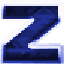#ACSUtils Wiki

An ACS library for ZDoom-based ports

# Rectangle API

This is a simple library that implements basic UI elements in ACS. This library is an addon for the cursor library.

ACSRect is not bundled with official ACSUtils. You can download it here.

Define `ACSUTILS_RECTLIB_SAVEDSTATES` to specify the amount of required rectangles.

## Usage

```...

#define ACSUTILS_RECTLIB_USERVARS 1 // user defined
#include "acsutils.acs"
#include "acsrect.acs"

...```

## Example

This script implements two simple buttons. You also can move them by using the right mouse button.

```script 1 (void) net clientside
{
int rects;
rects = RectCreate(0, 0, 64.0, 64.0);
rects = RectCreate(128.0, 128.0, 64.0, 64.0);

while(true)
{
UpdateCursor();
HudSetPoint(CursorX(), CursorY());
HudDrawText(1, "+");

for (int i = 0; i <= rects; i++)
{
if (RectIsGrabbed(i, BT_ALTATTACK))
RectFollowCursor(i);
if (RectIsPressed(i, BT_ATTACK))
log(s:"pressed");

for (int j = 0; j <= rects; j++)
{
if (RectIntersects(i, j))
log(s:"rect ", d:i, s:" intersects with ", d:j);
}

HudSetPoint(RectX1(i), RectX2(i));
HudDrawText(2+(i*4), "*");
HudSetPoint(RectX2(i), RectY1(i));
HudDrawText(3+(i*4), "*");
HudSetPoint(RectX1(i), RectY2(i));
HudDrawText(4+(i*4), "*");
HudSetPoint(RectX2(i), RectY2(i));
HudDrawText(5+(i*4), "*");
}

delay(1);
}
}   ```

# Functions

### RectCreate

`int RectCreate(fixed x, fixed y, fixed width, fixed height)`

Creates a rectangle on the stack with the given parameters. The result value is the rectangle's id in the stack.

### RectsDelete

`void RectsDelete(int start)`

Deletes a group of rectangles from stack, start == 0 will delete all rectangles. Use non-zero values to delete the rectangles only from the top of the stack.

## Rectangle coordinates

### RectX1

`fixed RectX1(int rect)`

Returns the X coordinate of rectangle vertexes №1, №3.

### RectY1

`fixed RectY1(int rect)`

Returns the Y coordinate of rectangle vertexes №1, №2.

### RectX2

`fixed RectX2(int rect)`

Returns the X coordinate of rectangle vertexes №2, №4.

### RectY2

`fixed RectY2(int rect)`

Returns the Y coordinate of rectangle vertexes №3, №4.

### RectCenterX

`fixed RectCenterX(int rect)`

Returns the X coordinate of the rectangle's center.

### RectCenterY

`fixed RectCenterY(int rect)`

Returns the Y coordinate of the rectangle's center.

### SetRectPosition

`void SetRectPosition(int rect, fixed x, fixed y)`

Sets the X Y of given rectangle. Rectangle position is its (X1; Y1) point.

### SetRectSize

`void SetRectSize(int rect, fixed width, fixed height)`

Changes the rectangle's size to width and height.

### RectWidth

`fixed RectWidth(int rect)`

Returns the width of the given rectangle.

### RectHeight

`fixed RectHeight(int rect)`

Returns the height of the given rectangle.

### RectFollowCursor

`void RectFollowCursor(int rect)`

Makes rect to follow mouse coordinates.

### RectIsPointInside

`bool RectIsPointInside(int rect, fixed x, fixed y)`

Returns true if the rectangle contains the point with the coordinates x y, otherwise returns false.

### RectIntersects

`bool RectIntersects(int rect, int rect2)`

Returns true if the rectangle with id rect intersects with other rectangle with id rect2.

Rectangle can't intersect with itself. So, if rect == rect2, this function returns false.

## User Data

You may also create custom user-defined rectangle properties. Define ACSUTILS_RECTLIB_USERVARS to specify the amount of these properties.

### SetRectUserData

`void SetRectUserData(int rect, int id, int value)`

Sets the value of the given custom property of the rectangle.

### RectGetUserData

`int RectGetUserData(int rect, int id)`

Returns the value of the rectangle's given custom property.

### Example

A simple implementation of a checkbox button.

```#define ACSRECT_USER_CHECKED 0

...

int checker = RectCreate(0, 0, 16, 16);

...

while(true)
{
...
int checked = RectGetUserData(checker, ACSRECT_USER_CHECKED);
if (RectIsClicked(checker, BT_ATTACK))
SetRectUserData(checker, ACSRECT_USER_CHECKED, !checked);
...

delay(1);
}```

## Rectangle action handlers

### RectIsHovered

`bool RectIsHovered(int rect)`

Returns true if the mouse cursor is inside the rectangle.

### RectIsClicked

`bool RectIsClicked(int rect, int key)`

Returns true if user clicked the rectangle once with key.

### RectIsDown

`bool RectIsDown(int rect, int key)`

Returns true if user is holding the key down and the mouse cursor is inside the rectangle.

### RectIsGrabbed

`bool RectIsGrabbed(int rect, int key)`

This function is similar to RectIsDown, but optimized for rectangle moving, because it saves the focus until user released the key.

### RectIsPressed

`bool RectIsPressed(int rect, int key)`

This function is similar to RectIsClicked, but unlike RectIsClicked it makes the rectangle work like a normal button:

• Returns true, if user released the key after clicking the rectangle.
• Returns true, if cursor went outside the rectangle after the user had held down the key.

Otherwise, it return false.